#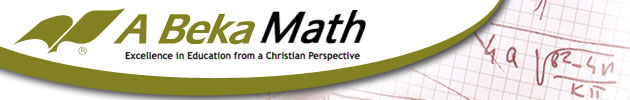Please Note: A Beka does not sell their materials to Exodus Books. The following overview is meant to help you evaluate A Beka as a curriculum, and give you some other options to consider as well.

A Beka's math program is a complete course for grades K-12. Comprehensive math programs can be hard to use because upper and lower level instruction are treated the same. A Beka's course develops with students. Those who use A Beka texts for student-directed work should know that the upper level math books require more teacher involvement, but there is plenty of support.

## How Do These Work?

Grades K-6 cover basic arithmetic. The kindergarten text focuses on counting, number recognition and simple addition, and from there students learn all the basics as well as fractions, decimals, basic geometry, and simple algebra. Some feel the elementary levels are too slow, but by sixth grade, students have learned everything they'd encounter in a comparable course. Each lesson includes lots of review. New topics are introduced thoroughly and reviewed often.

Grades 7-12 are less review-oriented. This is largely because the concepts build on each other, and too much backtrack would impede progress. The texts are faster moving, and you'll probably need to interact with your students more—you don't need to be a math genius yourself, though, since the teacher materials are clear and thorough.

There are a variety of materials for each level. At the core is the student worktext and answer key. K-2 are the only levels with a teacher guide; each student worktext page is reproduced in reduced size and includes teaching tips, lesson objectives, and notes. All other levels have an answer key, which is simply a reproduction of the student text with answers, and includes an appendix of teacher notes and objectives. Detailed plans for every lesson are in the curriculum book for each level—the plans walk you through preparation and in-class presentation.

Worktexts are in full color. The elementary texts have engaging illustrations; the junior high and high school texts are largely un-illustrated, though Consumer Mathematics and Plane Geometry have lots of photographs. Everything the student needs to know is in the student text—students read a portion of text, followed by plenty of examples and a problem set. While these texts are intended to be consumable workbooks, you could have your kids record their answers on a separate sheet of paper.

The teacher keys only include answers to problems. Solution keys are available for all upper level texts (anything after sixth grade) and include solutions for all in-text problems; test and quiz keys include solutions and answers. Each book includes an average of 170 lessons, one a day for a standard school year.

For each level there is a test/quiz book and a test/quiz key. Grades 1-6 include a consumable speed drills book and a speed drills key designed for review and reinforcement. There are no drill books for the older grades because there is plenty of review in the problem sets. For grades K-6 there are a variety of supplementary materials, from charts and flashcards to suggestions for math games. While these can be fun and even instructional, none of them are necessary for successful use of the course material.

The text for seventh grade is Basic Mathematics, largely a bridge review and introduction to basic concepts elaborated in later texts. Students exceptionally good at math could probably skip this level. Pre-Algebra, Algebra I, and Algebra II are the next three texts, followed by geometry. There are two choices for geometry. Plane Geometry is traditional Euclidean geometry with proofs; Analytic Geometry is a more modern approach based on the principles of algebra. The final book in the course is Trigonometry which provides students the basis for calculus and physics study.

There are two optional texts for high school students, Consumer Mathematics and Business Mathematics. These are intended to replace, not to be used simultaneously or consecutively, with the other texts. While students could work through both texts, it's probably best to choose one or the other. Consumer Mathematics deals with balancing a checkbook, budgeting and other elements of private economics, while Business Mathematics is more accounting-oriented and deals with similar issues from the corporate standpoint. These are useful texts if your student wants to pursue accounting or business studies, or if you don't see the need for learning advanced math.

## Our Honest Opinion:

A Beka is a long-time publisher of Christian curriculum. Their math program is the result of years of research and revision, reflected in its ease of use for both student and teacher. Like other A Beka subject courses, this one is equally adaptable for student or teacher directed use. If your child is good at math, handing him the text and grading his work will probably suffice; if he struggles, following the outlined lesson plans is likely the best choice.

The authors have adapted the material to suit each grade level, so students' ages and maturing learning styles are accommodated. If you plan to switch to or from A Beka, it's best to do so between sixth and seventh grade. If you want to continue following the incremental method after the elementary grades, Saxon would be a good choice. For the mastery-oriented style of the later texts, Singapore's Primary Math series would make good foundational texts in the early grades.

Review by C. Hollis Crossman
C. Hollis Crossman used to be a child. Now he's a husband and father who loves church, good food, and weird stuff. He might be a mythical creature, but he's definitely not a centaur. Read more of his reviews here.
Did you find this review helpful?
Parent Categories
A Beka Arithmetic & Mathematics
A Beka Arithmetic & Mathematics
Cathy Duffy Review
Reviews at HomeSchoolMath.net
117 Items found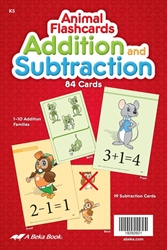Addition and Subtraction Animal Flashcards
from A Beka Books
for Kindergarten
in A Beka Arithmetic & Mathematics (Location: MAT-ABK)Addition Flashcards
from A Beka Books
in A Beka Arithmetic & Mathematics (Location: MAT-ABK)
\$16.00 (3 in stock)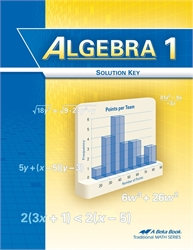Algebra 1 - Solution Key
3rd edition from A Beka Books
in A Beka Arithmetic & Mathematics (Location: MAT-ABK)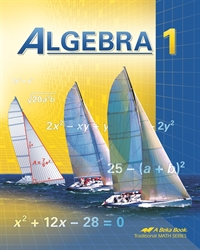Algebra 1 - Student Textbook
from A Beka Books
in A Beka Arithmetic & Mathematics (Location: MAT-ABK)Algebra 1 - Teacher Edition
3rd edition from A Beka Books
in A Beka Arithmetic & Mathematics (Location: MAT-ABK)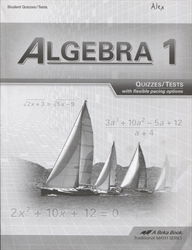Algebra 1 - Test/Quiz Book
3rd edition from A Beka Books
in A Beka Arithmetic & Mathematics (Location: MAT-ABK)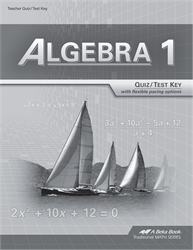Algebra 1 - Test/Quiz Key (with solutions)
3rd edition from A Beka Books
in A Beka Arithmetic & Mathematics (Location: MAT-ABK)
\$8.00 (1 in stock)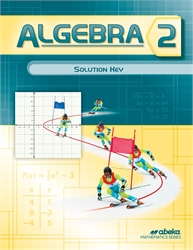Algebra 2 - Solution Key
from A Beka Books
in A Beka Arithmetic & Mathematics (Location: MAT-ABK)
\$46.50 (2 in stock)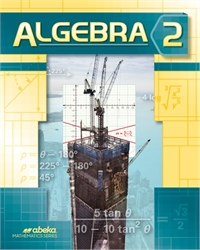Algebra 2 - Student Text
from A Beka Books
in A Beka Arithmetic & Mathematics (Location: MAT-ABK)
\$23.00 (2 in stock)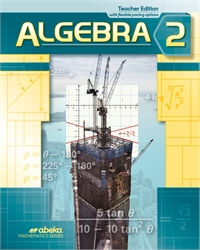Algebra 2 - Teacher Edition
from A Beka Books
in A Beka Arithmetic & Mathematics (Location: MAT-ABK)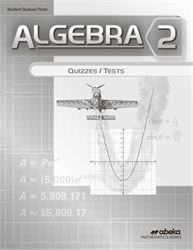Algebra 2 - Test/Quiz Book
from A Beka Books
in A Beka Arithmetic & Mathematics (Location: MAT-ABK)
\$7.00 (2 in stock)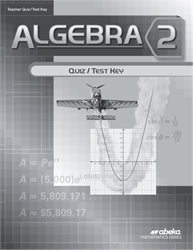Algebra 2 - Test/Quiz Key
from A Beka Books
in A Beka Arithmetic & Mathematics (Location: MAT-ABK)
\$12.50 (3 in stock)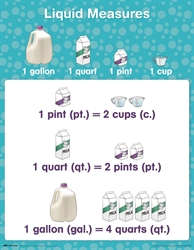Arithmetic 1 - Charts and Games
from A Beka Books
in A Beka Arithmetic & Mathematics (Location: MAT-ABK)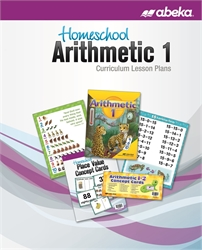Arithmetic 1 - Curriculum/Lesson Plans
3rd edition from A Beka Books
in A Beka Arithmetic & Mathematics (Location: MAT-ABK)
\$28.00 (1 in stock)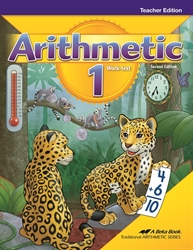Arithmetic 1 - Teacher Edition
from A Beka Books
in A Beka Arithmetic & Mathematics (Location: MAT-ABK)
\$14.50 (2 in stock)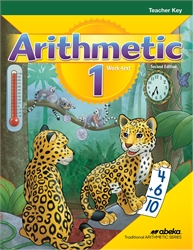Arithmetic 1 - Teacher Key
from A Beka Books
in A Beka Arithmetic & Mathematics (Location: MAT-ABK)
\$23.50 (3 in stock)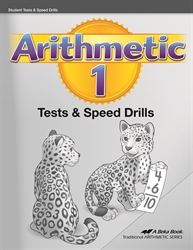Arithmetic 1 - Tests & Speed Drills
2nd edition from A Beka Books
in A Beka Arithmetic & Mathematics (Location: MAT-ABK)
\$7.00 (1 in stock)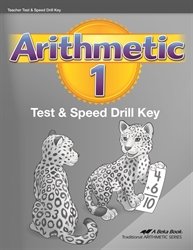Arithmetic 1 - Tests & Speed Drills Key
2nd edition from A Beka Books
in A Beka Arithmetic & Mathematics (Location: MAT-ABK)
\$13.00 (5 in stock)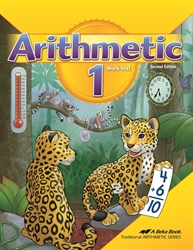Arithmetic 1 - Worktext
from A Beka Books
in A Beka Arithmetic & Mathematics (Location: MAT-ABK)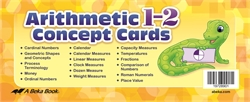Arithmetic 1-2 Concept Cards
from A Beka Books
in A Beka Arithmetic & Mathematics (Location: MAT-ABK)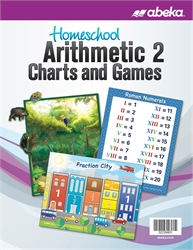Arithmetic 2 - Charts and Games
from A Beka Books
in A Beka Arithmetic & Mathematics (Location: MAT-ABK)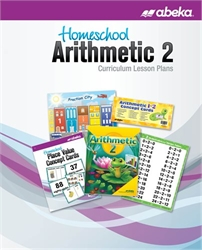Arithmetic 2 - Curriculum / Lesson Plans
from A Beka Books
in A Beka Arithmetic & Mathematics (Location: MAT-ABK)Arithmetic 2 - Student Tests/Speed Drills
2nd edition from A Beka Books
in A Beka Arithmetic & Mathematics (Location: MAT-ABK)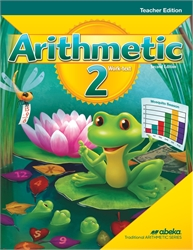Arithmetic 2 - Teacher Key
2nd edition from A Beka Books
in A Beka Arithmetic & Mathematics (Location: MAT-ABK)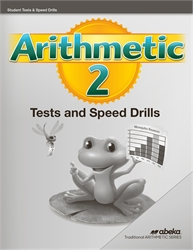Arithmetic 2 - Tests/Speed Drills
from A Beka Books
in A Beka Arithmetic & Mathematics (Location: MAT-ABK)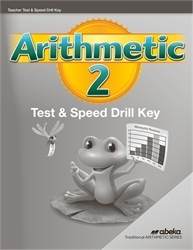Arithmetic 2 - Tests/Speed Drills Key
2nd edition from A Beka Books
in A Beka Arithmetic & Mathematics (Location: MAT-ABK)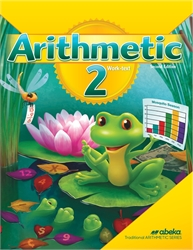Arithmetic 2 - Worktext
2nd edition from A Beka Books
in A Beka Arithmetic & Mathematics (Location: MAT-ABK)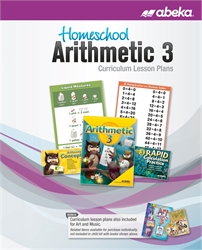Arithmetic 3 - Curriculum/Lesson Plans
6th edition from A Beka Books
in A Beka Arithmetic & Mathematics (Location: MAT-ABK)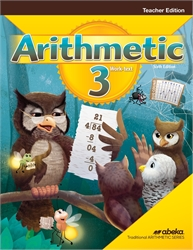Arithmetic 3 - Teacher Edition
6th edition from A Beka Books
in A Beka Arithmetic & Mathematics (Location: MAT-ABK)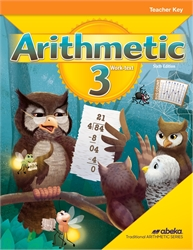Arithmetic 3 - Teacher Key
6th edition from A Beka Books
in A Beka Arithmetic & Mathematics (Location: MAT-ABK)
\$24.00 (1 in stock)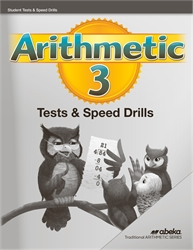Arithmetic 3 - Tests/Speed Drills
6th edition from A Beka Books
in A Beka Arithmetic & Mathematics (Location: MAT-ABK)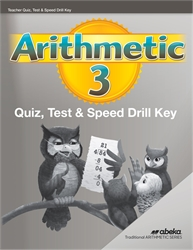Arithmetic 3 - Tests/Speed Drills Key
6th edition from A Beka Books
in A Beka Arithmetic & Mathematics (Location: MAT-ABK)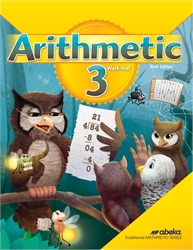Arithmetic 3 - Worktext
6th edition from A Beka Books
in A Beka Arithmetic & Mathematics (Location: MAT-ABK)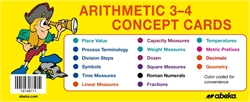Arithmetic 3-4 Concept Cards
from A Beka Books
in A Beka Arithmetic & Mathematics (Location: MAT-ABK)
\$18.00 (1 in stock)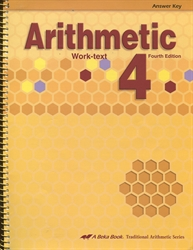Arithmetic 4 - Answer Key
4th edition from A Beka Books
in A Beka Arithmetic & Mathematics (Location: MAT-ABK)
\$14.00 (2 in stock)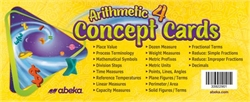Arithmetic 4 - Concept Cards
from A Beka Books
in A Beka Arithmetic & Mathematics (Location: MAT-ABK)Arithmetic 4 - Curriculum/Lesson Plans
from A Beka Books
in A Beka Arithmetic & Mathematics (Location: MAT-ABK)
\$21.00 (1 in stock)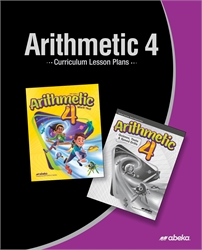Arithmetic 4 - Curriculum/Lesson Plans
from A Beka Books
in A Beka Arithmetic & Mathematics (Location: MAT-ABK)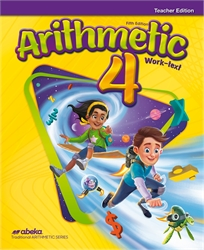Arithmetic 4 - Teacher Edition
5th edition from A Beka Books
in A Beka Arithmetic & Mathematics (Location: MAT-ABK)Arithmetic 4 - Tests/Speed Drills
from A Beka Books
in A Beka Arithmetic & Mathematics (Location: MAT-ABK)
\$5.00 (1 in stock)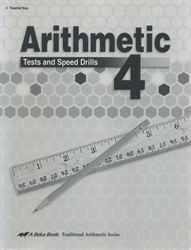Arithmetic 4 - Tests/Speed Drills Key
from A Beka Books
in A Beka Arithmetic & Mathematics (Location: MAT-ABK)
\$7.00 (4 in stock)Arithmetic 4 - Worktext
4th edition from A Beka Books
in A Beka Arithmetic & Mathematics (Location: MAT-ABK)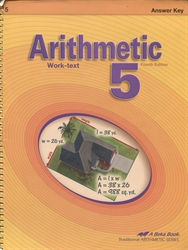Arithmetic 5 - Answer Key
4th edition from A Beka Books
in A Beka Arithmetic & Mathematics (Location: MAT-ABK)
\$17.00 (4 in stock)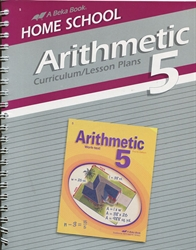Arithmetic 5 - Curriculum/Lesson Plans
from A Beka Books
in A Beka Arithmetic & Mathematics (Location: MAT-ABK)
\$21.00 (2 in stock)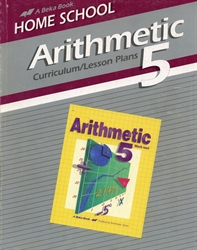Arithmetic 5 - Curriculum/Lesson Plans (old)
from A Beka Books
in A Beka Math (Old Versions only) (Location: OABK-MAT)
\$4.00 (1 in stock)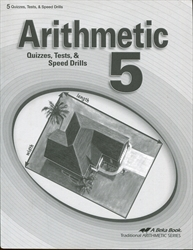Arithmetic 5 - Tests/Speed Drills
from A Beka Books
in A Beka Arithmetic & Mathematics (Location: MAT-ABK)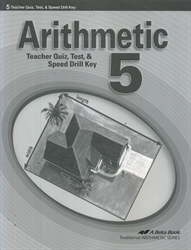Arithmetic 5 - Tests/Speed Drills Key
4th edition from A Beka Books
in A Beka Arithmetic & Mathematics (Location: MAT-ABK)
\$7.50 (2 in stock)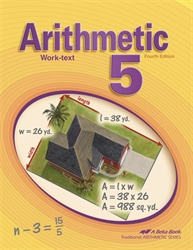Arithmetic 5 - Worktext
from A Beka Books
in A Beka Arithmetic & Mathematics (Location: MAT-ABK)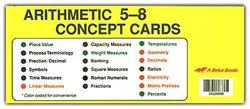Arithmetic 5-8 Concept Cards
from A Beka Books
in A Beka Arithmetic & Mathematics (Location: MAT-ABK)
\$26.00 (4 in stock)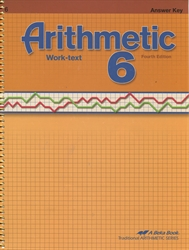Arithmetic 6 - Answer Key
4th edition from A Beka Books
in A Beka Arithmetic & Mathematics (Location: MAT-ABK)
\$17.00 (6 in stock)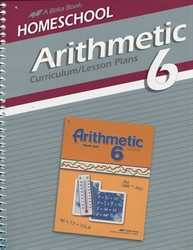Arithmetic 6 - Curriculum / Lesson Plans
from A Beka Books
in A Beka Arithmetic & Mathematics (Location: MAT-ABK)
\$21.50 (2 in stock)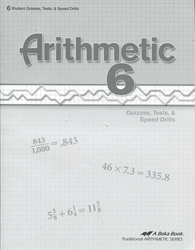Arithmetic 6 - Tests/Speed Drills
4th edition from A Beka Books
in A Beka Arithmetic & Mathematics (Location: MAT-ABK)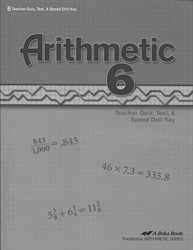Arithmetic 6 - Tests/Speed Drills Key
4th edition from A Beka Books
in A Beka Arithmetic & Mathematics (Location: MAT-ABK)
\$10.00 (4 in stock)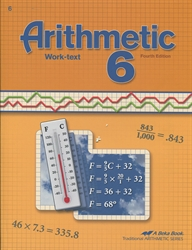Arithmetic 6 - Worktext
4th edition from A Beka Books
in A Beka Arithmetic & Mathematics (Location: MAT-ABK)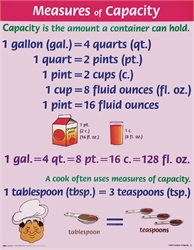Arithmetic Charts 3-8
5th edition from A Beka Books
in A Beka Arithmetic & Mathematics (Location: MAT-ABK)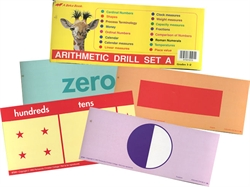Arithmetic Drill Set A (old)
from A Beka Books
in A Beka Math (Old Versions only) (Location: OABK-MAT)
\$12.00 (1 in stock)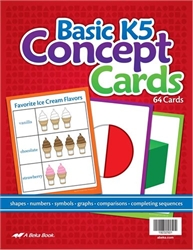Basic K5 Concept Cards
from A Beka Books
for Kindergarten
in A Beka Arithmetic & Mathematics (Location: MAT-ABK)
\$16.00 (1 in stock)
Basic K5 Concept Cards - Homeschool
from A Beka Books
for Kindergarten
in A Beka Arithmetic & Mathematics (Location: MAT-ABK)
\$7.00 (1 in stock)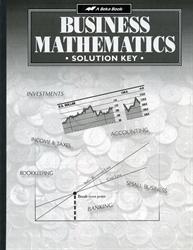Business Mathematics - Solution Key
2nd edition from A Beka Books
in A Beka Arithmetic & Mathematics (Location: MAT-ABK)
\$25.00 (1 in stock)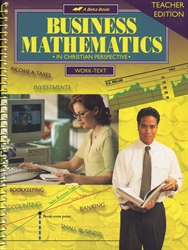Business Mathematics - Teacher Edition
2nd edition from A Beka Books
in A Beka Arithmetic & Mathematics (Location: MAT-ABK)
\$17.00 (1 in stock)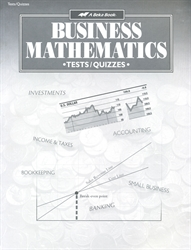Business Mathematics - Test/Quiz Book
2nd edition from A Beka Books
in A Beka Arithmetic & Mathematics (Location: MAT-ABK)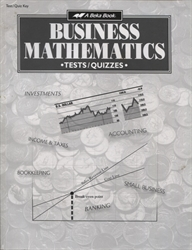Business Mathematics - Test/Quiz Key
2nd edition from A Beka Books
in A Beka Arithmetic & Mathematics (Location: MAT-ABK)
\$9.50 (2 in stock)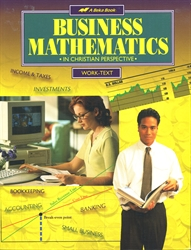Business Mathematics - Worktext
2nd edition from A Beka Books
in A Beka Arithmetic & Mathematics (Location: MAT-ABK)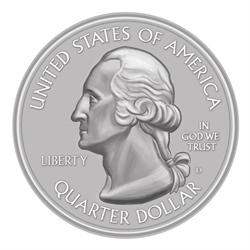Classroom Coins set
from A Beka Books
in A Beka Arithmetic & Mathematics (Location: MAT-ABK)
\$5.00 (3 in stock)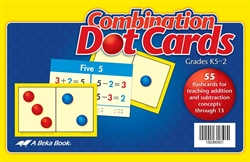Combination Dot Cards - K5 - 2
from A Beka Books
in A Beka Arithmetic & Mathematics (Location: MAT-ABK)
\$18.00 (2 in stock)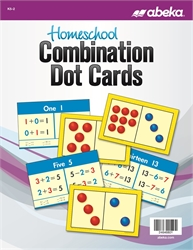Combination Dot Cards - K5 - 2 Homeschool
from A Beka Books
in A Beka Arithmetic & Mathematics (Location: MAT-ABK)
\$7.00 (1 in stock)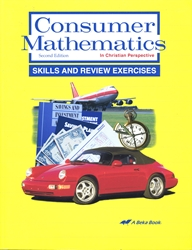Consumer Mathematics - Skills & Review Exercises
2nd edition from A Beka Books
in A Beka Arithmetic & Mathematics (Location: MAT-ABK)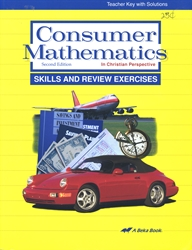Consumer Mathematics - Skills & Review Teacher Key
2nd edition from A Beka Books
in A Beka Arithmetic & Mathematics (Location: MAT-ABK)
\$18.00 (2 in stock)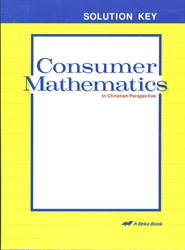Consumer Mathematics - Solution Key
from A Beka Books
in A Beka Arithmetic & Mathematics (Location: MAT-ABK)
\$25.00 (2 in stock)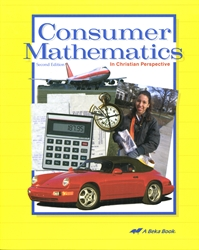Consumer Mathematics - Student Text
2nd edition from A Beka Books
in A Beka Arithmetic & Mathematics (Location: MAT-ABK)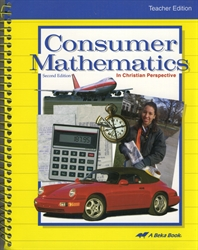Consumer Mathematics - Teacher Edition
2nd edition from A Beka Books
in A Beka Arithmetic & Mathematics (Location: MAT-ABK)
\$40.00 (1 in stock)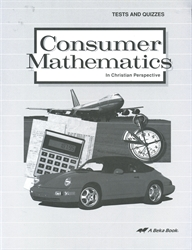Consumer Mathematics - Test/Quiz Book
2nd edition from A Beka Books
in A Beka Arithmetic & Mathematics (Location: MAT-ABK)
\$7.00 (1 in stock)
Consumer Mathematics - Test/Quiz Key
2nd edition from A Beka Books
in A Beka Arithmetic & Mathematics (Location: MAT-ABK)
\$9.50 (3 in stock)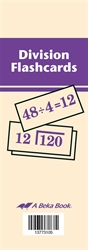Division Flashcards
from A Beka Books
in A Beka Arithmetic & Mathematics (Location: MAT-ABK)
\$15.00 (6 in stock)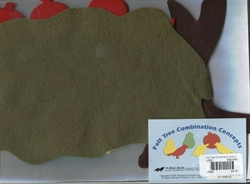Felt 3" Numerals
from A Beka Books
for Nursery-Kindergarten
in A Beka Arithmetic & Mathematics (Location: MAT-ABK)
\$8.00 (1 in stock)Homeschool Arithmetic 1 Charts and Games
from A Beka Books
in A Beka Arithmetic & Mathematics (Location: MAT-ABK)
\$11.50 (1 in stock)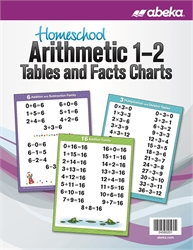Homeschool Arithmetic 1-2 Tables and Facts Charts
from A Beka Books
in A Beka Arithmetic & Mathematics (Location: MAT-ABK)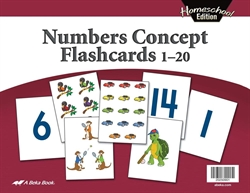Homeschool Number Concepts Flashcards
from A Beka Books
for Kindergarten Age 4
in A Beka Arithmetic & Mathematics (Location: MAT-ABK)
\$5.00 (1 in stock)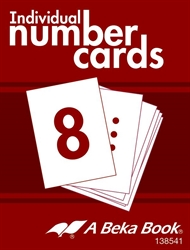Individual Number Cards
from A Beka Books
for Kindergarten
in A Beka Arithmetic & Mathematics (Location: MAT-ABK)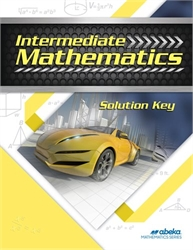Intermediate Mathematics - Solution Key
from A Beka Books
in A Beka Arithmetic & Mathematics (Location: MAT-ABK)
\$36.50 (1 in stock)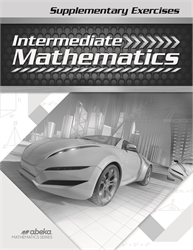Intermediate Mathematics - Supplementary Exercises
from A Beka Books
in A Beka Arithmetic & Mathematics (Location: MAT-ABK)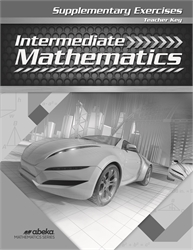Intermediate Mathematics - Supplementary Exercises Answer Key
from A Beka Books
in A Beka Arithmetic & Mathematics (Location: MAT-ABK)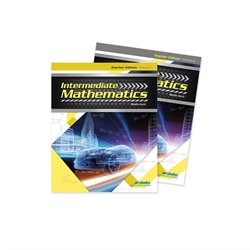Intermediate Mathematics - Teacher Edition (two volumes)
from A Beka Books
in A Beka Arithmetic & Mathematics (Location: MAT-ABK)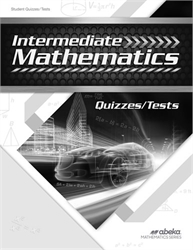Intermediate Mathematics - Test/Quiz Book
from A Beka Books
in A Beka Arithmetic & Mathematics (Location: MAT-ABK)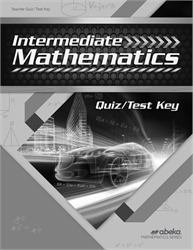Intermediate Mathematics - Test/Quiz Key
from A Beka Books
in A Beka Arithmetic & Mathematics (Location: MAT-ABK)
\$12.00 (2 in stock)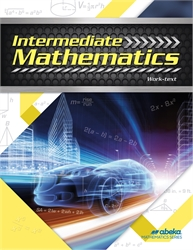Intermediate Mathematics - Worktext
4th edition from A Beka Books
in A Beka Arithmetic & Mathematics (Location: MAT-ABK)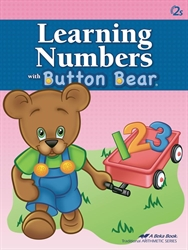Learning Numbers With Button Bear
2nd edition from A Beka Books
for 2-3 years
in A Beka Arithmetic & Mathematics (Location: MAT-ABK)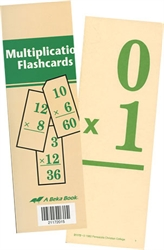Multiplication Flashcards
from A Beka Books
in A Beka Arithmetic & Mathematics (Location: MAT-ABK)
\$15.00 (1 in stock)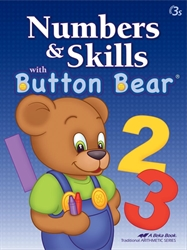Numbers and Skills with Button Bear
2nd edition from A Beka Books
for 2-3 years
in A Beka Arithmetic & Mathematics (Location: MAT-ABK)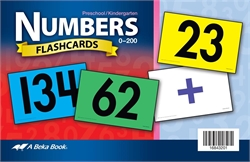Numbers Flashcards
2nd edition from A Beka Books
for Kindergarten
in A Beka Arithmetic & Mathematics (Location: MAT-ABK)
\$23.00 (4 in stock)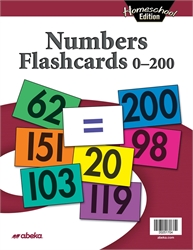Numbers Flashcards 0-200 - Homeschool
from A Beka Books
in A Beka Arithmetic & Mathematics (Location: MAT-ABK)
\$7.50 (3 in stock)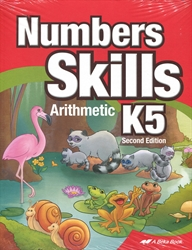Numbers Skills K5
from A Beka Books
for Kindergarten Age 5
in A Beka Arithmetic & Mathematics (Location: MAT-ABK)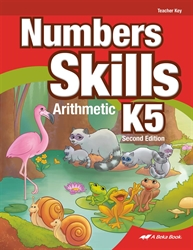Numbers Skills K5 - Teacher Key
2nd edition from A Beka Books
for Kindergarten Age 5
in A Beka Arithmetic & Mathematics (Location: MAT-ABK)
\$20.00 (1 in stock)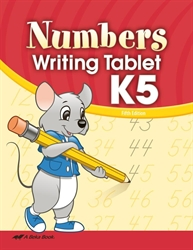Numbers Writing Tablet
from A Beka Books
for Kindergarten Age 5
in A Beka Arithmetic & Mathematics (Location: MAT-ABK)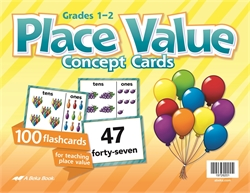Place Value Concept Cards
from A Beka Books
in A Beka Arithmetic & Mathematics (Location: MAT-ABK)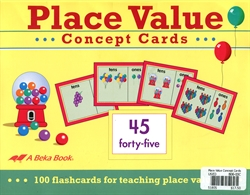Place Value Concept Cards (old)
from A Beka Books
in A Beka Arithmetic & Mathematics (Location: MAT-ABK)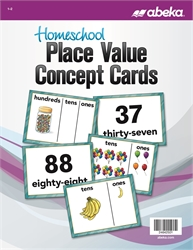Place Value Concept Cards - Homeschool
from A Beka Books
in A Beka Arithmetic & Mathematics (Location: MAT-ABK)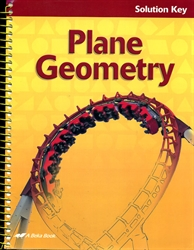Plane Geometry - Solution Key
2nd edition from A Beka Books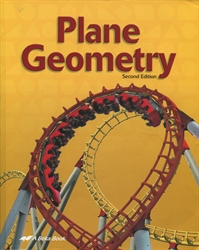Plane Geometry - Student Text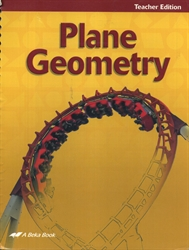Plane Geometry - Teacher Edition